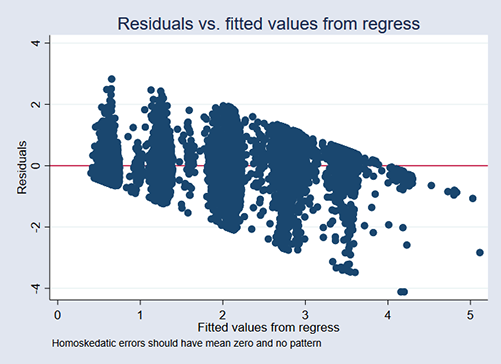»  Home »  Products »  Stata 15 »  Heteroskedastic linear regression

# Heteroskedastic linear regression

## Highlights

• Linear regression with multiplicative heteroskedastic errors
• Flexible exponential function for the variance
• Maximum likelihood estimator
• Two-step GLS estimator
• Support for Bayesian estimation
• Robust, cluster–robust, and bootstrap standard errors
• Complex survey designs support

hetregress fits linear regressions in which the variance is an exponential function of covariates that you specify. It allows you to model the heteroskedasticity. When we fit models using ordinary least squares (regress), we assume that the variance of the residuals is constant. If it is not constant, regress reports biased standard errors, leading to incorrect inferences. hetregress lets you deal with the heterogeneity.

Modeling the variance as an exponential function also produces more efficient parameter estimates if the variance model is correctly specified.

hetregress implements two estimators for the variance: a maximum likelihood (ML) estimator and a two-step GLS estimator. The ML estimates are more efficient than those obtained by the GLS estimator if the mean and variance function are correctly specified and the errors are normally distributed. The two-step GLS estimates are more robust if the variance function is incorrect or the errors are nonnormal.

## Let's see it work

We model students' high school performance (grade point average or GPA) as a function of

• their attendance rate (attend)
• whether they are freshmen, sophomores, juniors, or seniors
• their participation in sports (sports)
• their participation in after school activities
• whether they take advanced placement courses (ap)
• whether they are boys (boy)
• their parent's maximum level of educational attainment (pedu)

We could fit the model by typing

. regress  gpa  attend i.(grade sports extra ap boy pedu)


After fitting the model, we found evidence of heteroskedasticity using the existing postestimation command estat hettest, which did not surprise us. We suspected that the variance might increase with the student's grade level if nothing else. As students age, they become different. We had suspicions about the effects of other variables as well.So we refit the model using hetregress:

. hetregress gpa attend i.(grade sports extra ap boy pedu),

Heteroskedastic linear regression               Number of obs     =     10,000
ML estimation
Wald chi2(10)     =  499044.20
Log likelihood =  4658.587                      Prob > chi2       =     0.0000

gpa        Coef.   Std. Err.      z    P>|z|     [95% Conf. Interval]

gpa
attend     .7390506   .0082289    89.81   0.000     .7229222     .755179

sophomore      .2361749   .0014954   157.94   0.000      .233244    .2391058
junior      .7811304   .0028099   277.99   0.000     .7756231    .7866378
senior       1.27962    .003619   353.59   0.000     1.272527    1.286713

sports
yes      .3872983   .0025673   150.86   0.000     .3822666    .3923301

extra
yes      .3843422   .0040179    95.66   0.000     .3764673    .3922171

ap
yes      .2101632   .0073612    28.55   0.000     .1957354     .224591

boy
boy     -.4082246   .0015562  -262.32   0.000    -.4112748   -.4051744

pedu
college      1.599457   .0042422   377.04   0.000     1.591143    1.607772
graduate      3.070231   .0106216   289.06   0.000     3.049413    3.091049

_cons    -.0445178    .008372    -5.32   0.000    -.0609266   -.0281091

lnsigma2
sophomore      .1260056   .0437898     2.88   0.004     .0401793     .211832
junior      1.852363   .0487709    37.98   0.000     1.756774    1.947953
senior      2.476228   .0465629    53.18   0.000     2.384967     2.56749

pedu
college     -3.226682   .0324147   -99.54   0.000    -3.290214    -3.16315
graduate      .5139875   .0502405    10.23   0.000     .4155179     .612457

ap
yes      1.070959   .0918929    11.65   0.000     .8908526    1.251066

extra
yes      1.064171    .053584    19.86   0.000     .9591484    1.169194

ap#extra
yes#yes      .1559163   .3113822     0.50   0.617    -.4543816    .7662142

_cons     -3.53778   .0361415   -97.89   0.000    -3.608616   -3.466944

LR test of lnsigma2=0: chi2(8) = 7478.32                  Prob > chi2 = 0.0000

The coefficients under the heading gpa compose our main model for the mean of gpa.

The coefficients under the heading lnsigma2 are the coefficients of the exponential model for the variance.

The likelihood-ratio test reported at the bottom of the table tells us that our model of the variance fits the data better than a model where the variance is constant.